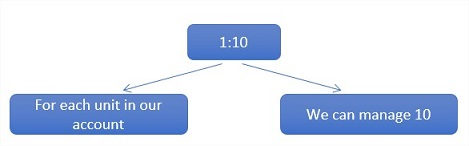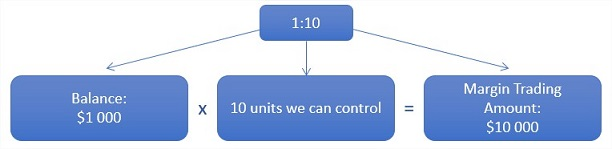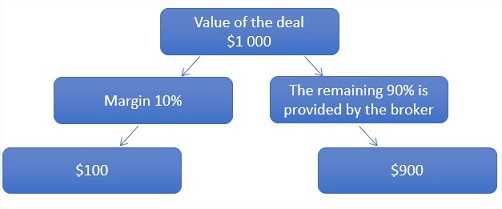# Margin and Leverage: How to use them effectively?

15.06.2021

Margin and leverage are two terms that are often misunderstood by beginner traders. The key to success in trading on financial markets is risk management, but it is only possible when we use the leverage effect effectively.

Margin trading can be used in a variety of markets, including the currency, commodity, futures, stock, and crypto markets. And its goal is to provide a higher return on investments, which makes this approach attractive to both traders with more capital and those with less.

### What is margin and leverage?

In fact, these two terms show the same thing, but in a different way. The difference is that leverage is presented as a ratio and margin as a percentage. This is what causes confusion in most beginners, which does not allow them to fully comprehend and understand.

#### Leverage

The leverage ratio is presented as follows:

 1:5 1:10 1:30 1:100

This ratio shows us how many units we can manage for each unit of our account balance. In other words, if we have an account balance of \$1 000 and leverage of 1:10, that means we can manage \$10 000.Why can we manage \$10 000 when we only have \$1 000 in our account? Because leverage of 1:10 means that for each unit in the account, we can control 10. Since we have \$1 000 units in the account, each of which allows us to control 10 others, the end result will be \$10 000.The ratio shows the maximum amount that we can manage at a certain level of leverage. The more leverage we have, the more money we can manage.

The easiest way to find the maximum amount we can trade is when we multiply the funds in our account by the number of units that leverage allows us to manage.

 Leverage 1:5 1:10 1:30 1:100 Balance \$1 000 \$1 000 \$1 000 \$1 000 Trading amount \$5 000* \$10 000 \$30 000 \$100 000

*1000x5=5000

#### Margin

The margin percentage shows what part of the total value of the deal we have to provide with our own funds. If our margin is 10% and the total value of the deal is \$1 000, we only need to provide \$100 of our own funds from the account balance in order to be able to open and maintain this position.When we multiply the value of the deal by the margin percentage - in this example \$1 000x10%, we will see that we only need to provide \$100 to open a position worth \$1 000.

Where does the other \$900 come from then? This amount is provided by the broker.

 Margin percentage 3.34% 5% 10% 20% Value of the deal \$1 000 \$1 000 \$1 000 \$1 000 Margin amount \$33.40* \$50 \$100 \$200

*1000*3.34%=33.40

### What is the connection between leverage and margin?

As mentioned above in the text, margin and leverage show the same thing. The reason is that each leverage ratio corresponds to a certain margin rate. To find this percentage, we need to divide the leverage ratio. Then we multiply by 100 and find the margin percent. For example, a leverage of 1:10 corresponds to a margin of 10%.

 Leverage 1:5 1:10 1:30 1:100 Margin 20%* 10% 3.34% 1%

*1:5=0.2; 0.2x100=20%

The greater leverage we have, the lower the margin rate will be. Conversely, the lower leverage means a higher margin.

### What is the connection with the risk?

There is a connection between the use of leverage and the risk in trading. The higher leverage leads to greater risk, which can have a negative impact on the balance, even with smaller price deviations.

Many traders do not realize that risk for the account can be too big not only from one larger position but also from many smaller trades if they pile up. For this reason, the total value of the open positions should be closely monitored, as margin trading can significantly increase your profits, but can also increase your realized losses.